# Average and Instantaneous Rate of Change

The average rate of change represents the total change in one variable in relation to the total change of another variable. Instantaneous rate of change, or derivative, measures the specific rate of change of one variable in relation to a specific, infinitesimally small change in the other variable. The average rate of change of a function can be determined with secant lines and the instantaneous rate of change can be determined with tangent lines. As you will learn, these rates can also be determined using a special type of math called calculus.

What is the rate of change?

Rate of change is the change in one variable in relation to the change in another variable. A common rate of change is speed, which measures the change in distance travelled in relation to the time elapsed. Olympic Gold Medalist, Usain Bolt, became the world’s fastest man running at a top speed of 44.72 km/hr during the 100-meter dash. On average, his speed was a bit slower (nonetheless, very impressive) at 37.58 km/hr.  Bolt’s top speed is an example of an instantaneous rate of change, and his average speed is an average rate of change.

### Average Rate of Change

Secant lines are found by connecting two points on a curve. The slope of the secant line between two points represents the average rate of change in that interval.

Formula:

Average Rate of Change = Slope(m) = △y/△x ==How to find the average rate of change between two points using a secant line:

Step 1: Draw a secant line connecting the two points.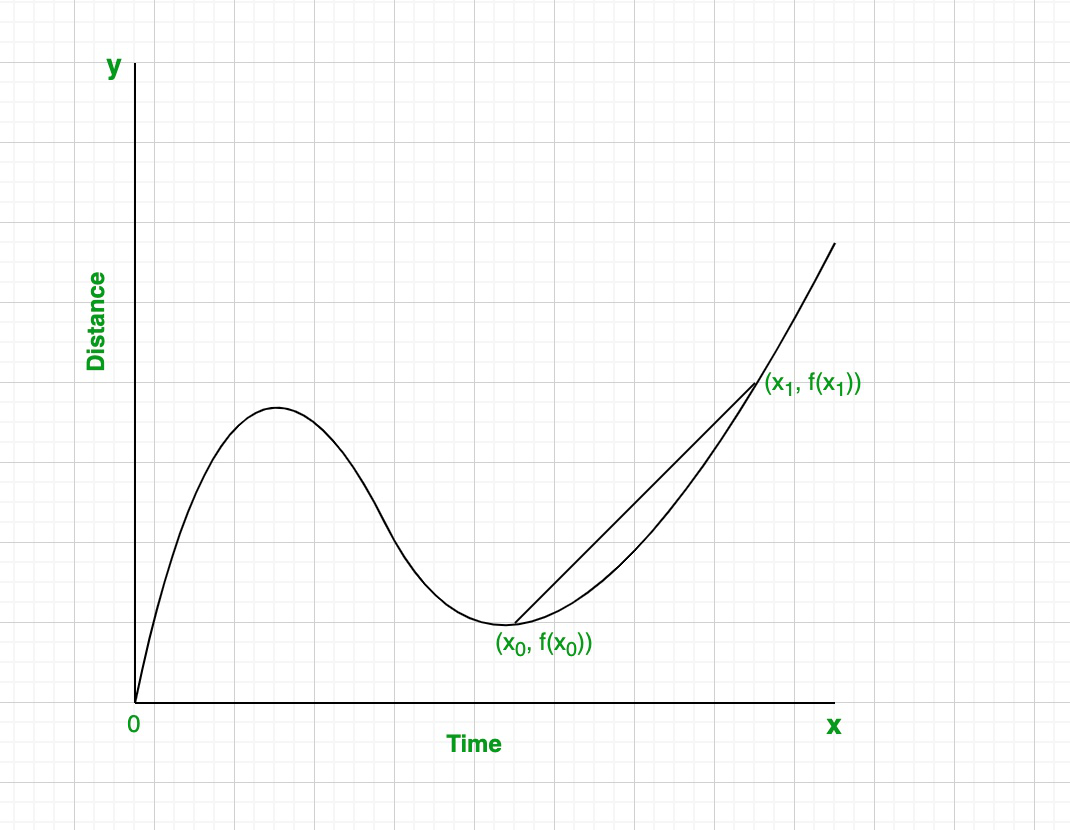Step 2: Use the coordinates of the two points to calculate the slope.

Equation of slope:
Slope =The average change of the function over the given time interval[x0, x1
Slope =The slope of the secant line represents the average
rate of change of the graph in that interval.

Once you’ve calculated the slope of the secant line, you use the slope can write an equation to represent it.

For Example:
Equation of slope:
y – y0 = m(x – x0

m = slope of secant line = 4/7
x0 = 2
y0 = 9Hence, the equation of the secant line between x = 2 and x = 9 is
y – 9 =### Derivatives (Instantaneous Rate of Change)

The tangent line at a point is found by drawing a straight line that touches a curve at that point without crossing over the curve. In other words, the line should locally touch only one point. The slope of the tangent line at a point represents the instantaneous rate of change, or derivative, at that point.

Formula:

Instantaneous Rate of Change =How to find the derivative at a point using a tangent line:

Step 1: Draw a tangent line at the point.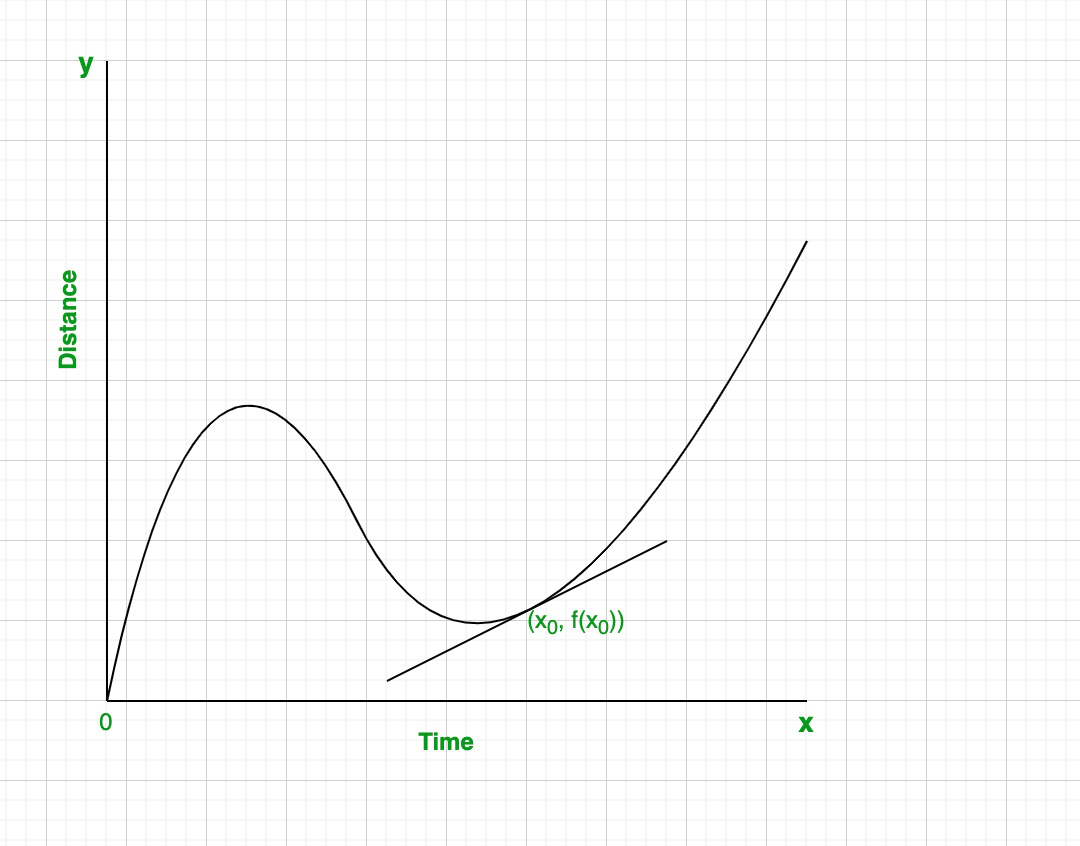Step 2: Use the coordinates of any two points on that line to calculate the slope.

Equation of slope:
Slope =The average change of the function over the given time interval x0
Slope =The slope of the tangent line at a point represents
the instantaneous rate of change, or derivative, at that point.

Once you’ve calculated the slope of the tangent line, you can write an equation to represent it.

For Example: Equation of slope:
y – y0 = m(x – x0

m = slope of tangent line =x0 = 16
y0 = 6Hence, the equation of the tangent line at x = 16 is
y – 16 = \frac{5}{6}(x – 6)

### Derivative Notation

In the early 18th century, there was controversy between the great mathematicians Isaac Newton and Gottfried Wilhelm Leibniz over who the first invent calculus. This argument became known as Prioritätsstreit, or “priority dispute” in German. The disagreement has had lasting impact on the mathematical world, leaving us with two standard derivative notations. Lagrange notation is another common derivative notation, established by French mathematician and philosopher, Joseph-Louis Lagrange

If we take the function y = f(x), So

1. Leibniz notation:

“First derivative of y with respect to x”“Second derivative of y with respect to x”“First derivative of y with respect to x
at x = 2″2. Newton notation:

The number of dots above the function
variable represents how many times the
function has been differentiated.
“First derivative of y”“Second derivative of y”“First derivative of y at x = 2”3. Lagrange notation:

The number of apostrophes after the function
variable represents how many times the
function has been differentiated.
“First derivative of y”
⇒y’
“First derivative of y at x = 2”
⇒y'(2)
“Second derivative of y”
⇒y”

4. Euler’s notation

“First derivative”
⇒ Dx
“Second derivative”
⇒ Dx2
Here, D represents the differential operator

### Sample Problems

Question 1. Find the average rate of change over the interval x = 4, x = 6.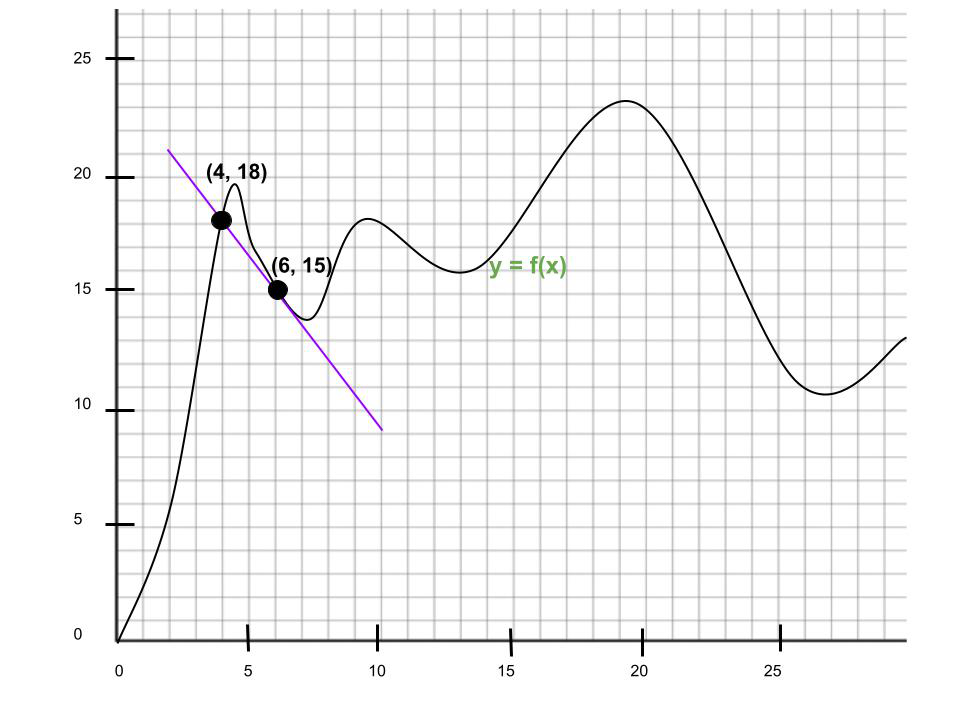Solution:

Point 1: (4, 18)

Point 2: (6, 15)

SlopeHence, the average rate of change =Question 2. Write the equation of the tangent line at x = 16.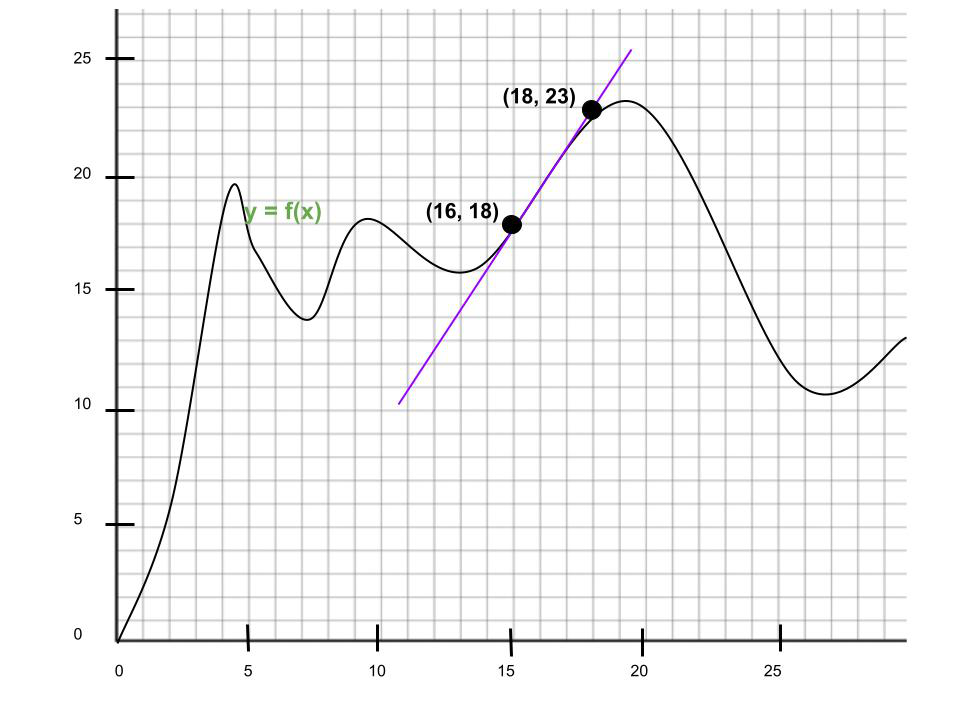Solution:

Point of intersection: (16, 18)

Slope =Equation of tangent line:Question 3. What is the derivative of the graph at x = 20? Express your answer in Leibniz notation.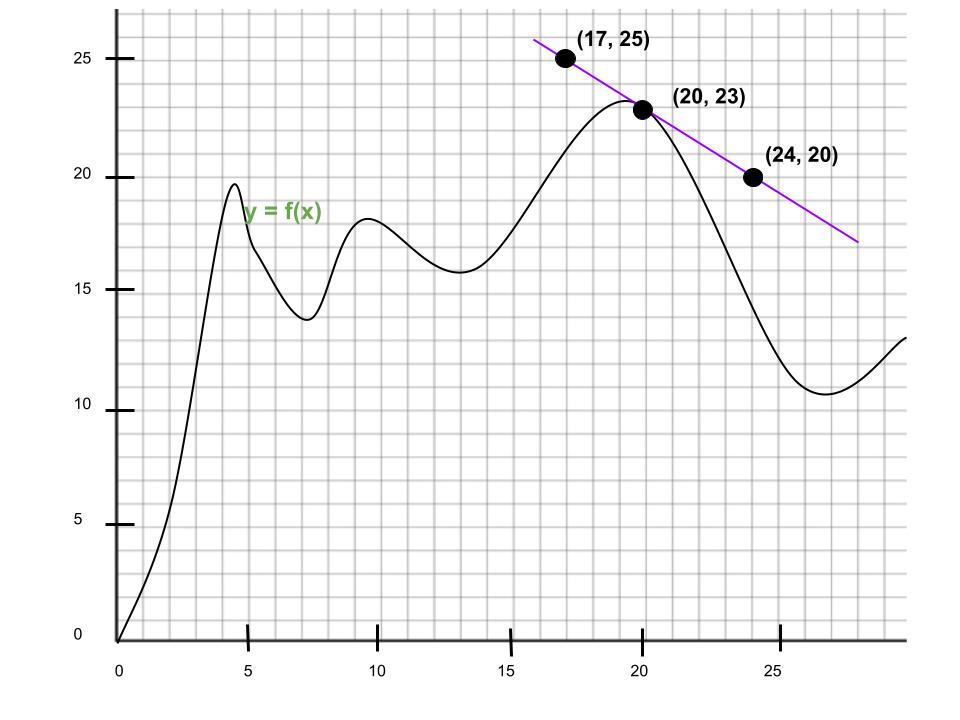Solution:

Given point of intersection: (20, 23)

Slope of tangent lineSo, the Leibniz notation:Question 4. Find the derivative of the graph at x = 4. Express your answer in Newton’s notation.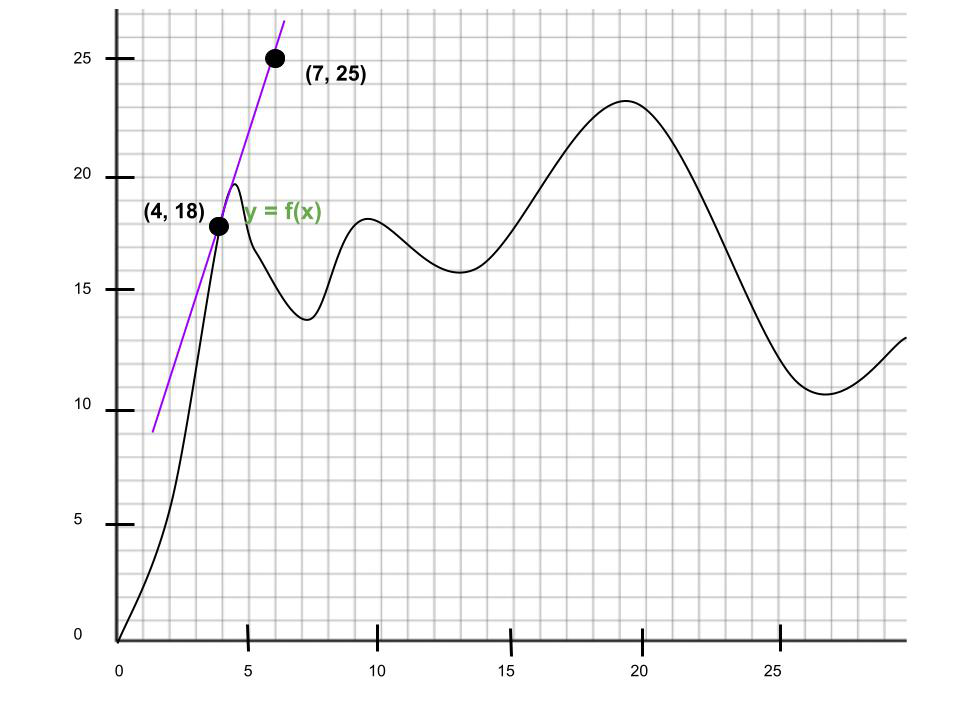Solution:

Given point of intersection: (4, 18)

Slope of tangent line =So, the Newton’s notationQuestion 5. Find the average rate of change over the interval x = 4, x = 25. How does this compare to the derivative at x = 4?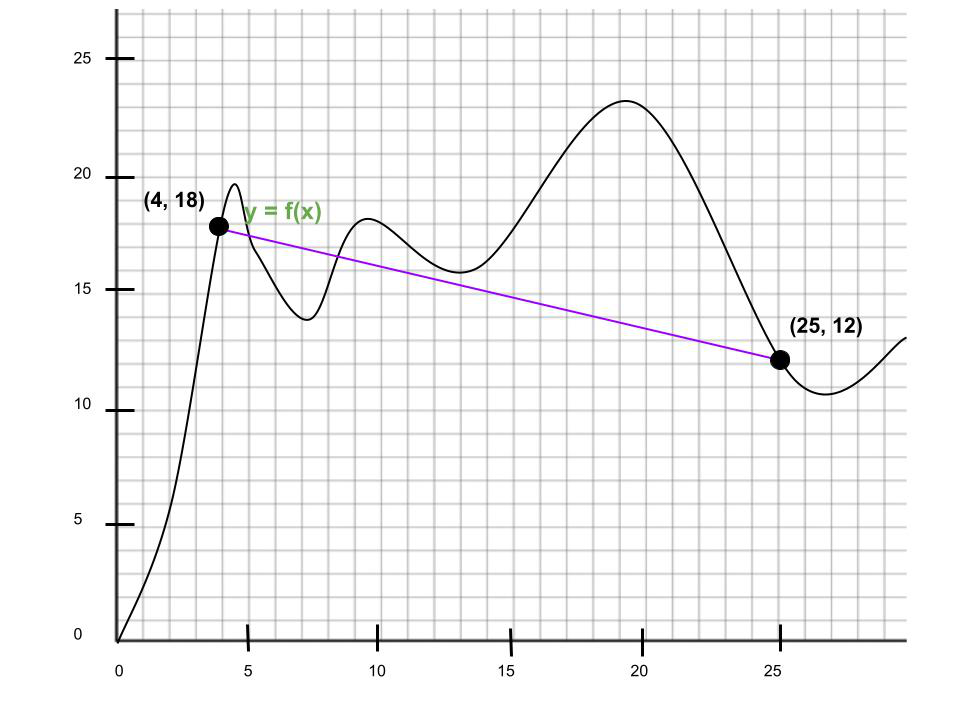Solution:

Slope of secant lineThe average rate of change over x = 4, x = 25 is -6/21,

which is less than the derivative of y at x = 4, which we found to

be 7/3.

Question 6. Find the instantaneous rate of change of the given function f(x) = 2x2 + 18 at x = 9 ?

Solution:

Given: f(x) = 2x2 + 18

f'(x) = 4x + 0

f'(x) = 4x

Now we have to find the instantaneous rate of change at x = 9

f(9) = 4x

f(9) = 4(9)

f(9) = 36

Question 7. Find the instantaneous rate of change of the given function f(x) = 4x2 + 12x + 8 at x = 4 ?

Solution:

Given: f(x) = 4x2 + 12x + 8

f'(x) = 8x + 12

Now we have to find the instantaneous rate of change at x = 4

f(4) = 8x + 12

f(4) = 8(4) + 12

f(4) = 44

Whether you're preparing for your first job interview or aiming to upskill in this ever-evolving tech landscape, GeeksforGeeks Courses are your key to success. We provide top-quality content at affordable prices, all geared towards accelerating your growth in a time-bound manner. Join the millions we've already empowered, and we're here to do the same for you. Don't miss out - check it out now!

Related Tutorials